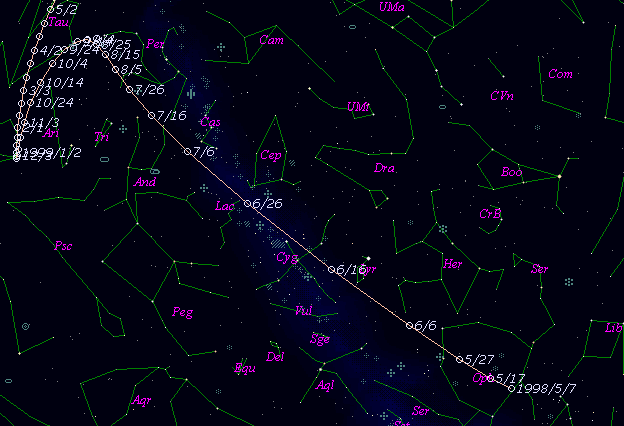# \$B%j%K%"WB@1(B

C/1998 K5 ( LINEAR )###\$B%W%m%U%#!<%k(B

 \$BH/8+F|(B 1998\$BG/(B5\$B7n(B26\$BF|(B \$BH/8+8wEY(B 18.0\$BEy(B \$BH/8+ Lincoln Laboratory Near-Earth Asteroid Research project

###\$B###\$B50F;MWAG(B

```   The following improved orbital elements by Kenji Muraoka, are
from 553 observations 1998 May 26 to 1999 Feb. 14, perturbations
by 9 Planets, Moon and 5 minor planets were taken into account.
The mean residual is +/- 0.60 arc seconds.

Epoch  =  1998 July  6.0  TT       JDT = 2451000.5
T  =  1998 July 17.44629       +/- 0.00002 (m.e.) TT
Peri. =   99.45875                +/- 0.00014
Node  =  211.11919                +/- 0.00014   (2000.0)
Incl. =    9.92700                +/- 0.00002
q  =    0.9635471              +/- 0.0000001 AU
e  =    0.9866924              +/- 0.0000040
1/a  =   +0.0138110              +/- 0.0000041 1/AU
( P  =    616 years )
```

###\$B@1?^(B###\$B8wEYJQ2=(B

```        m1 = 15.5 + 5 log\$B&\$(B + 25.0 log r  [  , 0]  (              \$B!A(B1998\$BG/(B 7\$B7n(B17\$BF|(B)
m1 = 14.5 + 5 log\$B&\$(B - 14.5 log r  [ 0,40]  (1998\$BG/(B 7\$B7n(B17\$BF|!A(B1998\$BG/(B 8\$B7n(B26\$BF|(B)
m1 = 14.0 + 5 log\$B&\$(B -  2.5 log r  [40,70]  (1998\$BG/(B 8\$B7n(B26\$BF|!A(B1998\$BG/(B 9\$B7n(B25\$BF|(B)
m1 = 12.7 + 5 log\$B&\$(B +  5.0 log r  [70,  ]  (1998\$BG/(B 9\$B7n(B25\$BF|!A(B              )

\$B"((B \$B3%?'\$N6J@~\$O!"(Bm1 = 15.5 + 5 log\$B&\$(B + 25.0 log r \$B\$N8wEY6J@~\$G\$9!#(B
```##### \$B50F;MWAG\$OB<2,7r<#;a\$N7W;;\$K\$h\$k\$b\$N\$G\$9!#(B \$B@1?^\$O(B StellaNavigator Ver.2.0 for Windows (\$B%"%9%H%m%"!<%D(B \$BJTCx(B / \$B%"%9%-!<=PHG6I4)(B) \$B\$G:n@.\$7\$?\$b\$N\$G\$9!#(B \$B8wEY%0%i%U\$O(BComet for Windows\$B\$G:n@.\$7\$?\$b\$N\$G\$9!#(B